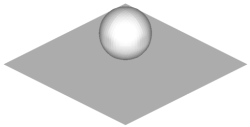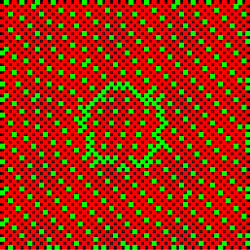# Parallel Algebraic Multigrid Methods (AMG)

#### Description

Multigrid methods (MG) are known to be optimal solvers for elliptic PDE’s, i.e. they only require $\mathcal{O}(N)$ computational work to solve the discretized system $Au=f$ up to given precision, where $N$ denotes the degrees of freedom. For many applications, however, it is difficult to construct a sequence of nested meshes required for geometric multigrid. In addition, the grids produced by standard coarsening (e.g. by doubling the mesh size) are not robust with respect to anisotropic problems or jumps in the PDE’s coefficients.
Algebraic Multigrid methods (AMG) overcome this problem. These methods first employ a setup phase to create a hierarchy of meshes, transfer and coarse grid operators using with information from the matrix A only. This hierarchy is used in the solution phase, i.e. the multigrid cycle.

While the parallelization of the solution phase is straightforward, this is not true for the setup phase. Especially the classical algorithm for the construction of the coarse grids is inherently sequential.
Different approaches to the parallelization have been proposed over the years. Some of them use the classical Ruge-Stüben coarsening scheme in the interior of each processor subdomain an a employ a special treatment at the boundaries to fix inconsistencies. Other algorithms employ parallel Maximal Independent Set techniques to create the coarse grid.

In our parallel coarsening scheme, the Coarse grid Classification (CGC) algorithm, we aim to keep the classical Ruge-Stüben coarsening scheme, which works excellent in the sequential case. Furthermore, we avoid inconsistencies at the subdomain boundaries instead of fixing them. We do so by first creating multiple candidate coarse grids on each processor subdomain. In a second stage, we assemble a consistent coarse grid, i.e. out of the candidate coarse grids we choose one coarse grid per processor subdomain such that they match at the interfaces.

#### Implementation

The CGC coarsening algorithm is included in two software packages.

#### Examples

Example: Flow simulation with density jumpThe above figure shows a drop of water falling through the air. We simulate this two-phase flow using a Chorin projection based flow solver. Each time step, we need to solve a Poisson equation in each time step, however, in this case, the density jump between air and water leads to a large condition number for the resulting linear system.
AMG methods discover this density jump and construct suitable coarse grids to provide an efficient preconditioner or solver. To reduce the memory overhead, a parallel AMG coarsening scheme should construct grids that stay as close as possible to the grid produced by the sequential algorithm. In the following, we present the three finest grids produced by the sequential algorithm (left) and by our parallel CGC method (right), where the domain is distributed on four processors.